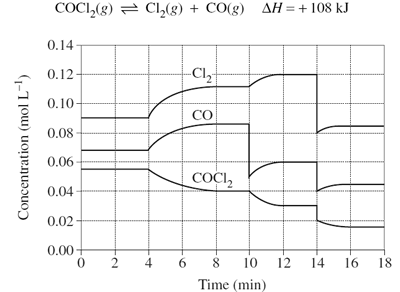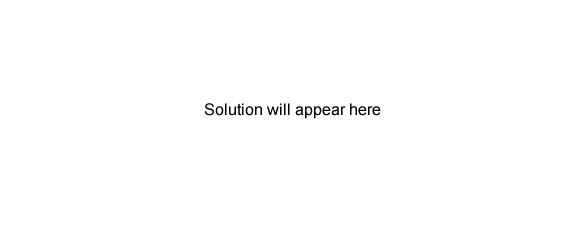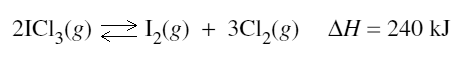Chemical equilibrium (2009 NSW HSC) 1)The graph shows the variation in concentration of reactant and products as a function of time for the following system.(i) Identify and explain each of the changes in conditions that have shaped the curves during the time the system was observed. Solution2) At a particular temperature, iodine trichloride dissociates into iodine gas and chlorine gas according to the following equation:Initially 0.35 mol of ICl3(g) was introduced into a 1.0 L container and allowed to come to equilibrium. At equilibrium there was 0.45 mol L–1 of Cl2(g). (i) Write the equilibrium constant expression for this reaction. Solution (ii) Calculate the value of K at this temperature. Solution (iii) What are TWO consequences of increasing the temperature of the mixture at equilibrium? Solution